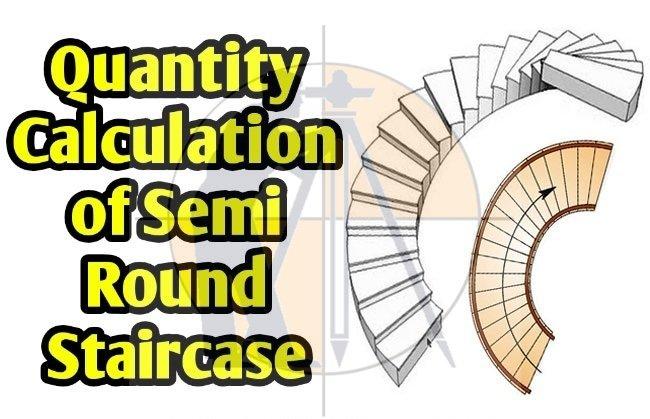Breaking News
Home / QA/QC Engineering / Quantity Calculation Of Round Staircase

# Quantity Calculation Of Round Staircase

## Quantity Calculation Of Round Staircase

This is a very useful article for Civil Engineering students. In this article, you will learn how to design and work out the quantity of semi round stairs.The calculation is done based on the following dimensions:-

Linear Length of Stair = ?

Inclined Length of Stair = ?

Waist of stair = 4.5 feet

Thickness of stair waist = 0.41 feet

Riser of stair = 6’’= 0.5 feet

Tread of stair = 1’’-3’’ -1’ (One side & opposite side)

No. of steps = Height / One Riser = 10 feet / 0.5 feet = 20 numbers

Now, it is required to determine the linear length as follow:-

2 (π x R) 0.50 = 2 (3.142 x 9.5) 0.50 = 29.84 feet

### How to Find Arc Length

Then it is required to determine the inclined length as follow:-

√(Base)2 + (Height)2

√(29.84)2 + (10)2

√(890.42) + (100) = √990.42 = 31.47 feet

So, inclined length = 31.47 feet

Then, you have to find out the waist volume by using the following formula:-

Waist Volume = Length x width x Thickness = 31.47 x 4.5 x 0.41 = 58.062 cft

Steps volume = ?

Width of tread = 1’- 3’’- 1’ = 1.25 + 1 = 2.25’/2 = 1.125’

### How To Calculate The Height Of Any Buildings And An Objects Using With Theodolite

Now, it is required to determine one step volume with the following formula:-

One step volume = L x W x H / 2 or Length x Width x Height / 2 (as the steps are in triangular shape)

= 4.5 x 1.125 x 0.5 / 2 = 1.26 cft

So, the volume of one step = 1.26

As the numbers of steps are 20

So, total steps = 20 x 1.26 = 25.2 cft

Total Volume = Volume of waist + Volume of total steps

= 58.062 + 25.2 = 83.26 cft

### THANKS.